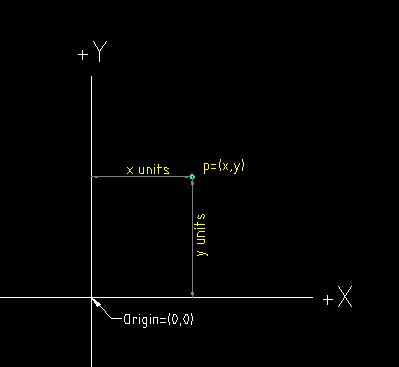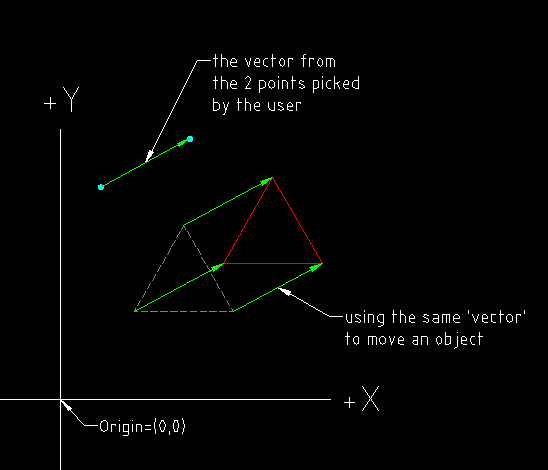### Understanding Vectors

Written by MickD www.dcservices.net.au

Vectors are very important to understand if you want to use them in your daily manipulations of computer graphics. They are one of the most fundamental building blocks of computational geometry and this will be the first of a few short articles about vectors where I will try to explain them in easy to understand terms. The articles will be about the the following:-
1. The difference between Points and Vectors and why we need them?
2. The components that make up a Vector
3. How we use them and where to next?
These articles won't be going into the nitty-gritty of the mathematics of vectors, that will come later. Like most things which are new to us, I think a simple explanation can go a long way to understanding what you're trying to achieve without getting too bogged down or lost in the math!

## Part 1 - Points and Vectors

### Points

Most of us are familiar with Points, in 2d (2 dimensions of space) we have a pair of coordinates usually written p(5,12) where 5 = 5 units along the x axis and 12 = 12 units along the y axis, all simple stuff I know but have a look at figure 1 and I'll get to the point shortly (pun intended!).Figure 1 - Showing a point in 2d space.
So, what else does a point have?
A point has a position in space and that's about it, but there are some things that we would like to know about that point such as what direction, or how far away is that point from another point? Enter the Vector -

### Vectors

A Vector is very similar to a Point and it has been argued that there is no difference when it comes to using them in computations as a vector is represented in a similar way to a point i.e. v(5,12), where 'v' denotes this array as a vector. But the 'great' mathematicians had more complex tasks in mind which only by developing the vector and it's mathematical functions could be solved. A simple problem, say you want to know in which direction or how far one point is from another, you can 'pull a vector' to it. How do you do that? have a look at the figure2 below.Figure 2 - Showing a point and a vector in 2d space.

This is the typical way to represent a vector, the end with the arrow is usually called the 'head' and the other end the 'tail'. Notice something else about this picture? the vector has a 'direction' and 'length' (also known as magnitude), have a look at figure 3 below.Figure 3 - Showing the length and direction of a vector.
Ok, so a vector at this stage doesn't seem like much help really as we could get the length and direction from the origin using simple trig, but what about from one point to another point out in space, what if we want to know the 'direction' from the point back to the origin! It also begs the question -

### Why else do we need Vectors?

In addition to what I mentioned above, there are many other things we can do with vectors that we just can't do with points, some are -
• Finding the distance from one point to another, or how far a point is perpendicular to a given line or object.
• Finding a 'direction' so we can move a graphical object in that direction ( we use a 'matrix' for this which is basically an array of vectors!)
• Finding the 'normal' of a plane ( a normal is a vector which is perpendicular to the plane, used for line/plane intersections and rendering algorithms ).
• Finding what side of a line a point is on (this deals with half-spaces and is handy for 'point in polygon' and 'point in volume' tests!)

### Ok, just a taste!

I haven't covered a great deal of area with this article and I did that deliberately so I didn't wander off and get us all lost in math and explanations, but I just have to show you a concept which is one of the reasons why vectors are so useful, have a look at figure 4 -Figure 4 - Showing how we can use a single vector to move an object.

### That's a wrap!

In the next article I will expand a little on this concept of vectors and break them up into their components to get a real understanding of what they're made of . I'll also give a brief explanation of the different types of calculations and their uses, nothing too deep, just enough to see where you would probably want to use them and a few diagrams showing what they do. My hope is that you will better understand the math behind them, there are plenty of examples out there already, they're just not too clear to the new-comer who hasn't just left college and majored in calculus!
If you are getting something out of this topic and you want to see more, leave a comment below . This is a huge topic, and writing about it in article form is going to be a huge task so I'll approach it like eating an elephant...one bite at a time! So please bare with me and I'll get them in as soon as I can, your positive feedback will definitely help!  ;)

Need drafting work?
We are having great success with Freelancer.com. With this website we can win all types of drafting work. People from all over the world are looking for freelancers skilled in AutoCAD, Revit, Civil 3D and Microstation, are you one of those people? If so you can live the freelancer lifestyle! and work from any location you want too. Freelancer.com, it works really well - we like it - and we believe you will too.